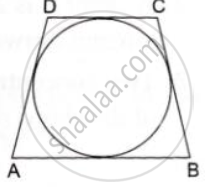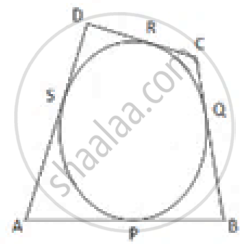# In the Given Figure, a Circle Touches All the Four Sides of a Quadrilateral Abcd Whose Three Sides Are Ab = 6cm, Bc=7cm and Cd=4 Cm. Find Ad. - Mathematics

In the given figure, a circle touches all the four sides of a quadrilateral ABCD whose three sides are AB = 6cm, BC=7cm and CD=4 cm. Find AD.#### SolutionLet the circle touch the sides of the quadrilateral AB, BC, CD and DA at P, Q, R and S respectively.

Given, AB = 6cm, BC = 7 cm and CD = 4cm.
Tangents drawn from an external point are equal.
∴ AP = AS, BP = BQ,CR = CQ and DR = DS
Now, AB + CD (AP + BP) + (CR + DR)

⇒AB + CD= (AS+ BQ) + (CQ+ DS)

⇒ AB +CD +(AS+ DS) +(BQ+ CQ)
∴ The length of AD is 3 cm.

Concept: Concept of Circle - Centre, Radius, Diameter, Arc, Sector, Chord, Segment, Semicircle, Circumference, Interior and Exterior, Concentric Circles
Is there an error in this question or solution?